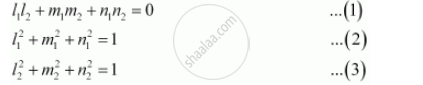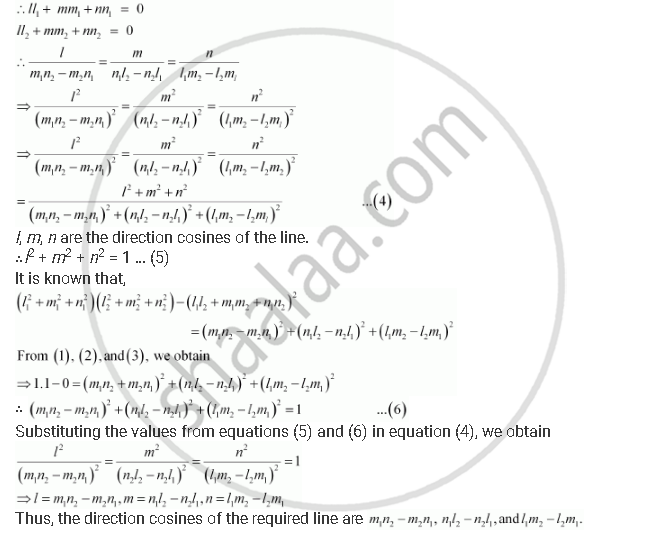Share

# If L1, M1, N1 And L2, M2, N2 Are the Direction Cosines of Two Mutually Perpendicular Lines, Show that the Direction Cosines of the Line Perpendicular to Both of These Are M1n2 − M2n1, N1l2 − N2l1, L1m2 ­− L2m1. - CBSE (Commerce) Class 12 - Mathematics

ConceptDirection Cosines and Direction Ratios of a Line

#### Question

If l1m1n1 and l2m2n2 are the direction cosines of two mutually perpendicular lines, show that the direction cosines of the line perpendicular to both of these are m1n2 − m2n1n1l2 − n2l1l1m2 ­− l2m1.

#### Solution

It is given that l1m1n1 and l2m2n2 are the direction cosines of two mutually perpendicular lines. Therefore,Let lmn be the direction cosines of the line which is perpendicular to the line with direction cosines l1m1n1 and l2m2n2Is there an error in this question or solution?

#### APPEARS IN

NCERT Solution for Mathematics Textbook for Class 12 (2018 to Current)
Chapter 11: Three Dimensional Geometry
Q: 2 | Page no. 497

#### Video TutorialsVIEW ALL 

Solution If L1, M1, N1 And L2, M2, N2 Are the Direction Cosines of Two Mutually Perpendicular Lines, Show that the Direction Cosines of the Line Perpendicular to Both of These Are M1n2 − M2n1, N1l2 − N2l1, L1m2 ­− L2m1. Concept: Direction Cosines and Direction Ratios of a Line.
S